全球高性能轮胎品牌——通伊欧轮胎TOYO TIRES，新年伊始正式推出了专为中国道路环境研发的静音性及舒适性轮胎通伊欧PROXES Comfort MC1。该款轮胎兼具优异的静音性和良好的舒适性，同时针对轿车及SUV车型分别开发设计了两款花纹。为了更直观深入地展现通伊欧PROXES Comfort MC1的卓越性能，通伊欧轮胎在位于上海世博园区的博大汽车公园特别设置了不同环节的试驾体验，与专业汽车媒体和来自全国的通伊欧轮胎渠道伙伴共聚一堂，零距离体验PROXES Comfort MC1轮胎带来的极致操控。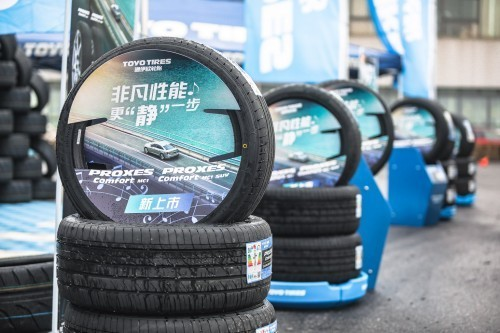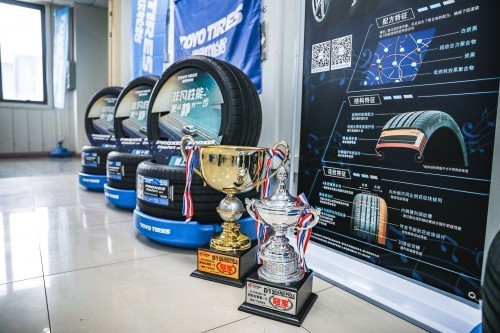据悉，通伊欧PROXES Comfort MC1新品是目前通伊欧投入市场的产品中运用最多科技的一款轮胎。PROXES Comfort MC1利用纳米平衡技术，在胎面配方中增加了二氧化硅的使用量，低燃耗效果加抓地力聚合物配方，在确保轮胎同时拥有低滚动阻力的同时还具备高速湿地刹车性能两大优点；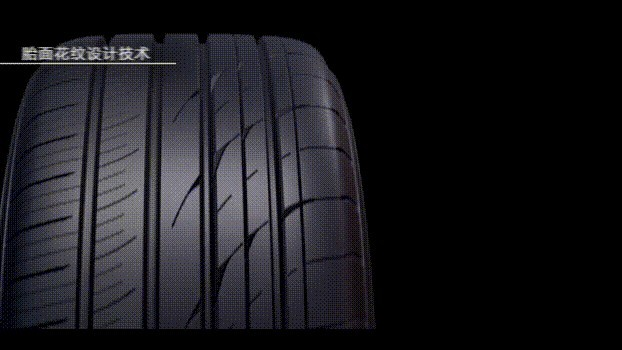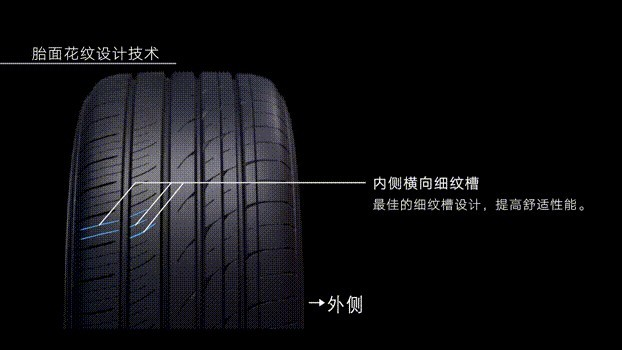复杂的胎面花纹设计，包括四条宽幅主沟槽，三连锥形与圆周方向花纹条和内外两侧3D波纹切槽设计，以及内外侧不同比例的花纹块排列、内侧横向细纹槽、外侧可变节距的花纹块和独特的静音壁设计，对提升轮胎的湿地性能、耐偏磨性以及静音效果都具有显著成效；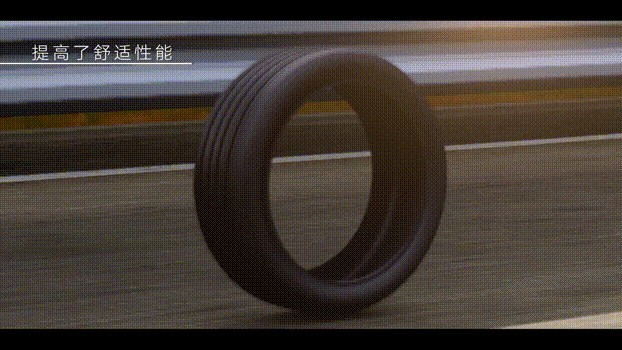通伊欧研发的高精度新工艺技术——正圆新技术的运用，实现了轮胎的正圆性和均一性，提高了车辆的驾乘舒适感。在众多黑科技的聚合之下，使得PROXES Comfort MC1成功获得中国轮胎标签认证，滚动噪声低至68分贝。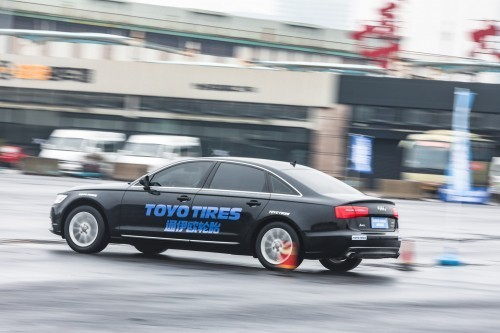在专业测试项目上，嘉宾分别体验了通伊欧静音舒适高性能轿车轮胎PROXES Comfort MC1的干湿地制动性能和高速S绕桩的操控表现。在湿地性能测试中，搭载了PROXES Comfort MC1的测试车能够沿着既定路线稳定行驶，得益于胎面花纹的排水设计，轮胎在湿地路面仍能保持较高的抓地力，在快速转向时也不会出现打滑。在湿地制动测试中，PROXES Comfort MC1在100-0km/h的刹车表现优异，湿地制动距离相比以往品缩短了9%。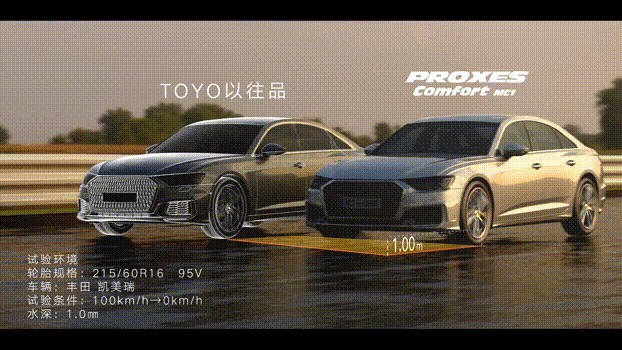在干地性能测试中，PROXES Comfort MC1直线加速起步稳定不打滑，100-0km/h干地制动距离相比以往品缩短了2%。种种表现都说明通伊欧在不断进行自我突破与自我迭代。高速S绕桩测试主要考验轮胎的干地抓地力和侧向支撑力。PROXES Comfort MC1轮胎在绕桩和紧急变向等动作中表现得游刃有余，即使每个桩桶的间距仅有3-4米，在强大的离心力之下，轮胎胎面依旧稳稳贴紧地面，运行轨迹始终可控。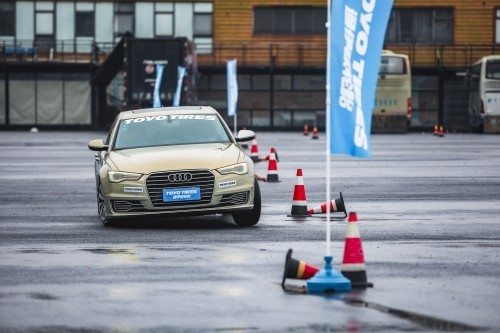消费者对驾乘舒适性的追求不断提高，不可否认噪音很大一部分来源于车辆行驶过程中发出的胎噪，而汽车厂家和轮胎厂家一直致力于达到性能和舒适的平衡。通伊欧静音舒适高性能轿车轮胎PROXES Comfort MC1不仅通过复杂的胎面花纹设计，以及模拟手枪消音器原理的独特静音壁设计，达到有效分散噪音、降低噪声的目的，而且在胎体内部也有静音设计，无接头带束层保护层，提高轮胎高速耐久性； 噪音缓冲层，大幅降低路面噪音。轮胎内外相辅相成的静音设计使得PROXES Comfort MC1的滚动噪声低至68分贝，而低于70分贝均是人耳可接受的舒适范围，68分贝也远低于上海市2018年底颁布并实施的客车噪音不得超过80分贝的交通噪音治理方案要求。在现场测试中，PROXES Comfort MC1的实际静音性能也得到了在场嘉宾的一致赞誉。除此之外，作为全球漂移界“一哥”的通伊欧轮胎，更是带来了顶级漂移赛事D1GP冠军法宝——通伊欧R888R高性能运动胎。在漂移试乘环节中，通过R888R与宝马E46的完美配合，为在场嘉宾留下了一次难忘的漂移极致体验。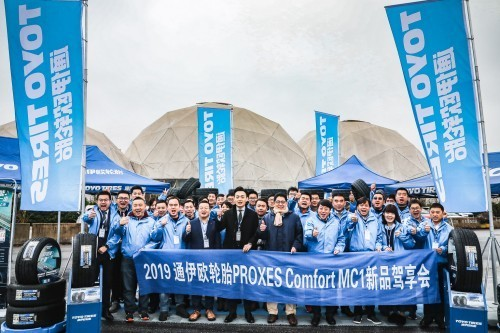通过本次驾享会，通伊欧展现出的不仅是两款优异的轮胎产品，更是从赛道到公路，从竞技到生活的品牌态度。同时通伊欧承诺中国车主，将凭借丰富的赛事经验和先进的工艺流程管理，不断为中国消费者研制出更高性能的民用轮胎产品，为广大城市车主带来堪比赛道的极致驾乘体验。

【关于通伊欧轮胎】

通伊欧轮胎（上海）贸易有限公司作为TOYO TIRE株式会社在中国的销售公司，成立于2002年11月。注册资金300万美元。TOYO TIRE投资比例为60%，三菱商事投资比例为40%。公司致力于轿车子午胎和载重子午胎在中国的推广，把日本高技术和优品质的通伊欧轮胎介绍给中国的广大用户。

##### 相关资讯

|分享

•已阅！握爪
•我手滑为你点赞
•128个赞！
•不明觉厉
•阅后既醉
•有钱！任性
•照片太美，我不敢看
•干货！杠杠的
•高大上
•膜拜中！
•请接受我的膝盖
•猫哥/猫妹么么哒
•神吐槽
•我只笑笑不说话
•我想静静
•窒息
##### 资讯相关车系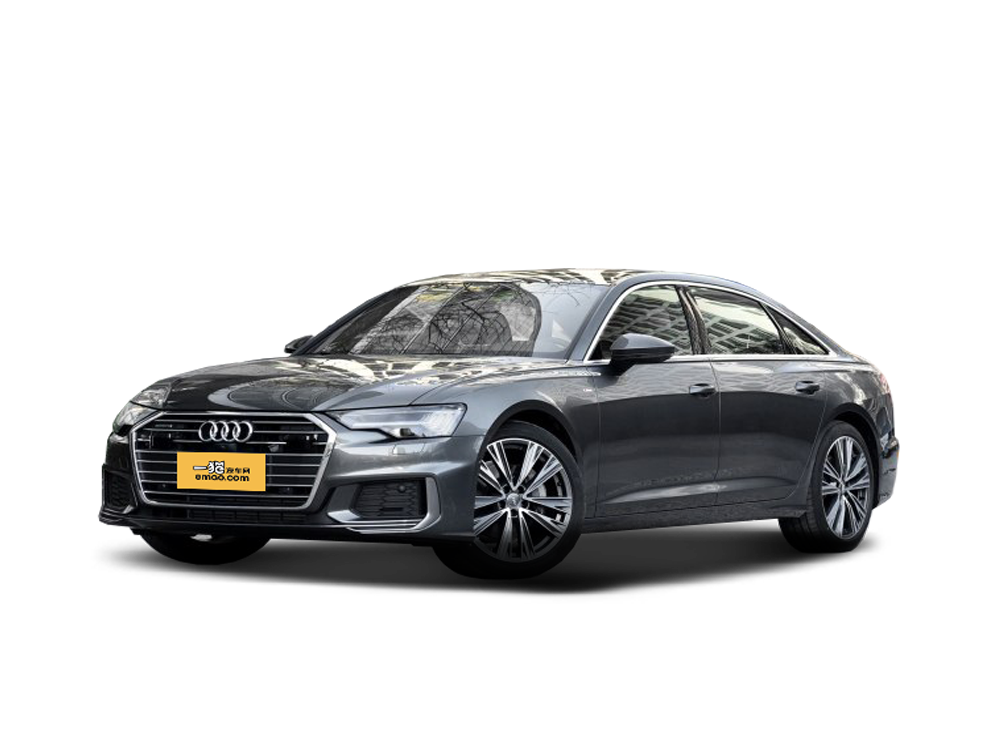##### 热度排行• 阿斯顿·马丁
• 奥迪
• 阿尔法罗密欧
• ALPINA

• 巴博斯
• 宝骏
• 宝马
• 保时捷
• 北汽制造
• 奔驰
• 奔腾
• 本田
• 比亚迪
• 标致
• 别克
• 宾利
• 布加迪
• 北汽威旺
• 北京
• 北汽绅宝
• 北汽幻速
• 北汽新能源
• 宝沃
• 比速汽车
• 北汽道达

• 昌河
• 长安
• 长城
• 长安商用
• 成功汽车
• 长江EV
• 长安轻车型

• 大众
• 道奇
• 东风
• 东风风神
• 东风小康
• 东南
• DS
• 东风风行
• 东风风度
• 东风风光

• 法拉利
• 菲亚特
• 丰田
• 福迪
• 福特
• 福田汽车
• 福汽启腾
• 风诺

• GMC
• 广汽传祺
• 广汽吉奥
• 观致

• 哈飞
• 海格
• 海马
• 华泰
• 黄海
• 恒天
• 红旗
• 哈弗
• 华颂
• 华凯
• 华泰新能源
• 汉腾汽车

• Jeep
• 江淮
• 江铃
• 捷豹
• 金杯
• 九龙
• 吉利汽车
• 金旅
• 金龙
• 江铃集团轻汽
• 江铃集团新能源
• 君马汽车
• 捷途

• 开瑞
• 凯迪拉克
• 科尼赛克
• 克莱斯勒
• KTM
• 卡威
• 凯翼
• 康迪
• 康迪电动汽车集团

• 猎豹汽车
• 兰博基尼
• 劳斯莱斯
• 雷克萨斯
• 雷诺
• 理念
• 力帆
• 莲花汽车
• 林肯
• 铃木
• 陆风
• 路虎
• 路特斯
• 领志
• 领克
• 零跑汽车

• MG
• MINI
• 马自达
• 玛莎拉蒂
• 迈凯伦
• 摩根

• 纳智捷

• 讴歌
• 欧宝
• 欧朗
• 欧拉
• 欧尚汽车

• 帕加尼

• 奇瑞
• 启辰
• 起亚
• 前途
• 庆铃汽车

• 日产
• 荣威
• 瑞麒汽车
• 如虎
• 瑞驰

• 上汽大通
• smart
• 三菱
• 双环
• 双龙
• 斯巴鲁
• 斯柯达
• 萨博
• 思铭
• 赛麟
• SWM斯威汽车

• TESLA
• 泰卡特
• 腾势

• 威麟
• 威兹曼
• 沃尔沃
• 五菱汽车
• 五十铃
• 潍柴英致
• WEY
• 蔚来
• 威马汽车

• 现代
• 雪佛兰
• 雪铁龙
• 西雅特
• 新特汽车
• 小鹏汽车

• 野马汽车
• 一汽
• 依维柯
• 英菲尼迪
• 永源
• 驭胜

• 中华
• 中兴
• 众泰
• 知豆
• 之诺
• 正道汽车
• A
• B
• C
• D
• E
• F
• G
• H
• I
• J
• K
• L
• M
• N
• O
• P
• Q
• R
• S
• T
• U
• V
• W
• X
• Y
• Z

• 阿斯顿·马丁
• 奥迪
• 阿尔法罗密欧
• ALPINA

• 巴博斯
• 宝骏
• 宝马
• 保时捷
• 北汽制造
• 奔驰
• 奔腾
• 本田
• 比亚迪
• 标致
• 别克
• 宾利
• 布加迪
• 北汽威旺
• 北京
• 北汽绅宝
• 北汽幻速
• 北汽新能源
• 宝沃
• 比速汽车
• 北汽道达

• 昌河
• 长安
• 长城
• 长安商用
• 成功汽车
• 长江EV
• 长安轻车型

• 大众
• 道奇
• 东风
• 东风风神
• 东风小康
• 东南
• DS
• 东风风行
• 东风风度
• 东风风光

• 法拉利
• 菲亚特
• 丰田
• 福迪
• 福特
• 福田汽车
• 福汽启腾
• 风诺

• GMC
• 广汽传祺
• 广汽吉奥
• 观致

• 哈飞
• 海格
• 海马
• 华泰
• 黄海
• 恒天
• 红旗
• 哈弗
• 华颂
• 华凯
• 华泰新能源
• 汉腾汽车

• Jeep
• 江淮
• 江铃
• 捷豹
• 金杯
• 九龙
• 吉利汽车
• 金旅
• 金龙
• 江铃集团轻汽
• 江铃集团新能源
• 君马汽车
• 捷途

• 开瑞
• 凯迪拉克
• 科尼赛克
• 克莱斯勒
• KTM
• 卡威
• 凯翼
• 康迪
• 康迪电动汽车集团

• 猎豹汽车
• 兰博基尼
• 劳斯莱斯
• 雷克萨斯
• 雷诺
• 理念
• 力帆
• 莲花汽车
• 林肯
• 铃木
• 陆风
• 路虎
• 路特斯
• 领志
• 领克
• 零跑汽车

• MG
• MINI
• 马自达
• 玛莎拉蒂
• 迈凯伦
• 摩根

• 纳智捷

• 讴歌
• 欧宝
• 欧朗
• 欧拉
• 欧尚汽车

• 帕加尼

• 奇瑞
• 启辰
• 起亚
• 前途
• 庆铃汽车

• 日产
• 荣威
• 瑞麒汽车
• 如虎
• 瑞驰

• 上汽大通
• smart
• 三菱
• 双环
• 双龙
• 斯巴鲁
• 斯柯达
• 萨博
• 思铭
• 赛麟
• SWM斯威汽车

• TESLA
• 泰卡特
• 腾势

• 威麟
• 威兹曼
• 沃尔沃
• 五菱汽车
• 五十铃
• 潍柴英致
• WEY
• 蔚来
• 威马汽车

• 现代
• 雪佛兰
• 雪铁龙
• 西雅特
• 新特汽车
• 小鹏汽车

• 野马汽车
• 一汽
• 依维柯
• 英菲尼迪
• 永源
• 驭胜

• 中华
• 中兴
• 众泰
• 知豆
• 之诺
• 正道汽车
• A
• B
• C
• D
• E
• F
• G
• H
• I
• J
• K
• L
• M
• N
• O
• P
• Q
• R
• S
• T
• U
• V
• W
• X
• Y
• Z

• 阿斯顿·马丁
• 奥迪
• 阿尔法罗密欧
• ALPINA

• 巴博斯
• 宝骏
• 宝马
• 保时捷
• 北汽制造
• 奔驰
• 奔腾
• 本田
• 比亚迪
• 标致
• 别克
• 宾利
• 布加迪
• 北汽威旺
• 北京
• 北汽绅宝
• 北汽幻速
• 北汽新能源
• 宝沃
• 比速汽车
• 北汽道达

• 昌河
• 长安
• 长城
• 长安商用
• 成功汽车
• 长江EV
• 长安轻车型

• 大众
• 道奇
• 东风
• 东风风神
• 东风小康
• 东南
• DS
• 东风风行
• 东风风度
• 东风风光

• 法拉利
• 菲亚特
• 丰田
• 福迪
• 福特
• 福田汽车
• 福汽启腾
• 风诺

• GMC
• 广汽传祺
• 广汽吉奥
• 观致

• 哈飞
• 海格
• 海马
• 华泰
• 黄海
• 恒天
• 红旗
• 哈弗
• 华颂
• 华凯
• 华泰新能源
• 汉腾汽车

• Jeep
• 江淮
• 江铃
• 捷豹
• 金杯
• 九龙
• 吉利汽车
• 金旅
• 金龙
• 江铃集团轻汽
• 江铃集团新能源
• 君马汽车
• 捷途

• 开瑞
• 凯迪拉克
• 科尼赛克
• 克莱斯勒
• KTM
• 卡威
• 凯翼
• 康迪
• 康迪电动汽车集团

• 猎豹汽车
• 兰博基尼
• 劳斯莱斯
• 雷克萨斯
• 雷诺
• 理念
• 力帆
• 莲花汽车
• 林肯
• 铃木
• 陆风
• 路虎
• 路特斯
• 领志
• 领克
• 零跑汽车

• MG
• MINI
• 马自达
• 玛莎拉蒂
• 迈凯伦
• 摩根

• 纳智捷

• 讴歌
• 欧宝
• 欧朗
• 欧拉
• 欧尚汽车

• 帕加尼

• 奇瑞
• 启辰
• 起亚
• 前途
• 庆铃汽车

• 日产
• 荣威
• 瑞麒汽车
• 如虎
• 瑞驰

• 上汽大通
• smart
• 三菱
• 双环
• 双龙
• 斯巴鲁
• 斯柯达
• 萨博
• 思铭
• 赛麟
• SWM斯威汽车

• TESLA
• 泰卡特
• 腾势

• 威麟
• 威兹曼
• 沃尔沃
• 五菱汽车
• 五十铃
• 潍柴英致
• WEY
• 蔚来
• 威马汽车

• 现代
• 雪佛兰
• 雪铁龙
• 西雅特
• 新特汽车
• 小鹏汽车

• 野马汽车
• 一汽
• 依维柯
• 英菲尼迪
• 永源
• 驭胜

• 中华
• 中兴
• 众泰
• 知豆
• 之诺
• 正道汽车
• A
• B
• C
• D
• E
• F
• G
• H
• I
• J
• K
• L
• M
• N
• O
• P
• Q
• R
• S
• T
• U
• V
• W
• X
• Y
• Z